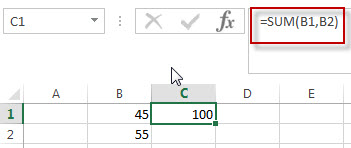# SUM Function in Excel

This post will guide you how to use Excel SUM function with syntax and examples in Microsoft excel.

## Description

The Excel SUM function will adds all numbers in a range of cells and returns the sum of these values. You can add individual values, cell references or ranges in excel.

The SUM function is a build-in function in Microsoft Excel and it is categorized as a Math and Trigonometry Function.

The SUM function is available in Excel 2016, Excel 2013, Excel 2010, Excel 2007, Excel 2003, Excel XP, Excel 2000, Excel 2011 for Mac.

## Syntax

The syntax of the SUM function is as below:

`= SUM(number1,[number2],…)`

Where the SUM function arguments are:

• number1 -This is a required argument. A numeric value that you want to sum.
• [number2],…– This is an optional argument.  Numbers that you want to find the sum of.

Note:

• The SUM function can accept up to 255 arguments

Excel SUM Function Examples

The below examples will show you how to use Excel SUM Function to return the sum of a set of numbers.

#1 To get the sum value of Cell B1 and B2, just using the following excel formula:

`=SUM(B1,B2)`### Related Functions

• Excel SUMIF Function
The Excel SUMIF function sum the numbers in the range of cells that meet a single criteria that you specify. The syntax of the SUMIF function is as below:=SUMIF (range, criteria, [sum_range])…
• Excel SUMIFS Function
The Excel SUMIFS function sum the numbers in the range of cells that meet a single or multiple criteria that you specify. The syntax of the SUMIFS function is as below:=SUMIFS (sum_range, criteria_range1, criteria1, [criteria_range2, criteria2], …)…
• Excel SUMSQ Function
The Excel SUMSQ function returns the sum of squares of a given set of arguments.The syntax of the SUMSQ function is as below:=SUMSQ(number1, [number2], …)…
• Excel SUMX2MY2 Function
The Excel SUMX2MY2 function returns the sum of the difference of squares between two supplied arrays.The syntax of the SUMX2MY2 function is as below:=SUMX2MY2 (array_x, array_y)…

### Excel SUM Function Examples

• Count Cells That Contain Specific Text
This post will discuss that how to count the number of cells that contain specific text or certain text in a specified cells of range in Excel. How to get the total number of cells that contain certain text.……
• Sum Same Cell Range on Multiple Worksheets
This post will explains that how to use the Excel 3-D reference to reference the same cell or a range of cells on multiple worksheets, then try to sum the same cell range from multiple worksheet with an Excel formula..……
• Using Data Validation to Limit Calculated Cell
This post will explains that how to use the Excel 3-D reference to reference the same cell or a range of cells on multiple worksheets, then try to sum the same cell range from multiple worksheet with an Excel formula..……
• Sort Cells by Specific word or words
how to sort cells or text values in a column based on a specific word even if the word is in the text string in the cell. How do I sort cells in a column by the number of a specific word or words in excel……
• Limit Formula Result to Maximum or Minimum Value
You can use the MIN function or Max function to create a formula to limit a number within a minimum or maximum value. .….
• Sum Specific Row or Column in Named Range
Assuming that you have a name range “excelhow”, if you want to sum a specific row (row 2) in named range, you need to create a formula based on the SUM function and the OFFSET function and the COLUMNS function to achieve the reuslt….
• Show Only Positive Values
You can create a formula based on the IF function, and the SUM function to sum all values in the range A1:C5 and just show only positive values…
• COUNTIF with Multiple Criteria
To count the number based on multiple criteria in the same range or column in Excel, you can create a formula based on the SUMPRODUCT function and the COUNTIF function.….
• Sum Multiple Columns based on One Criteria
You need to create a formula based on the SUM function to sum sale values for all two months in a newly helper column. And then create another formula based on the SUMIF function to sum total values for product “excel”. ……
• Sum Every Nth Row or Column
If you want to sum every nth rows in Excel, you can create an Excel Array formula based on the SUM function, the MOD function and the ROW function..….
• Count Dates in Given Year/Month/Day in Excel
You can create a formula based on the SUMPRODUCT function and the YEAR function to count dates by a give year….
• Sum the Absolute Values
Assuming that you have a list of data in range B1:B6 that contain negative numbers and positive numbers, and you want to sum all absolute values in these range of cells, You can use a formula based on the SUMIF function to achieve the result….